#Function Repository Resource:

# SymbolicSystem

Get the evolution of a symbolic system

Contributed by: Abigail Nussey
 ResourceFunction["SymbolicSystem"][rule,init,t] generates a list representing the evolution of the symbolic system with the specified rule from initial condition init for t steps.

## Details and Options

Some interesting rules are: e[x_][y_]x[y][e[y]], e[x_][y_]x[x[y]], e[x_][y_]e[x][y], e[x_][y_]x[e[y]][x], e[x_][y_]x[y[x]], e[x_][y_]x[y[e][e]], e[x_][y_]e[x[e][y[e]]], e[x_][y_]e[y[e[e][e]][x]].

## Examples

### Basic Examples

Sample evolution of a symbolic system:

 In:=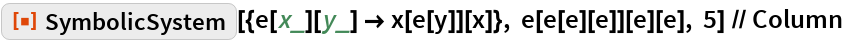Out=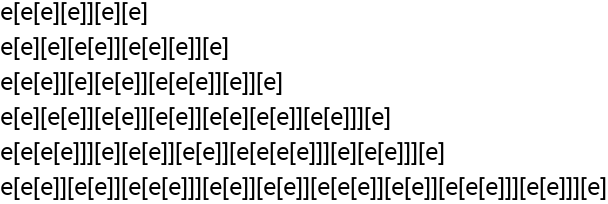Define the auxiliary function ToBrackets:

 In:=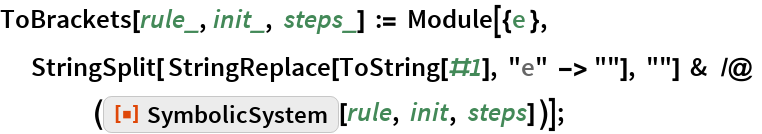In:=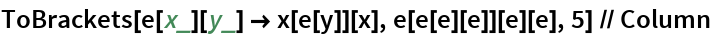Out=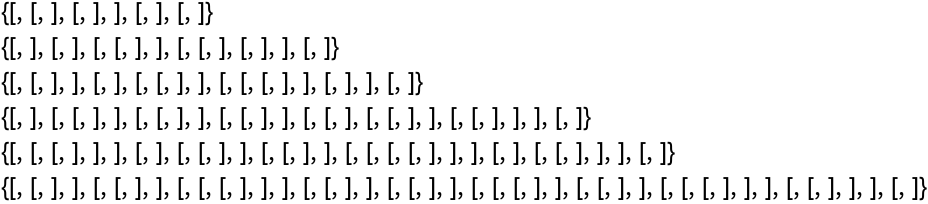Steps versus step lengths:

 In:=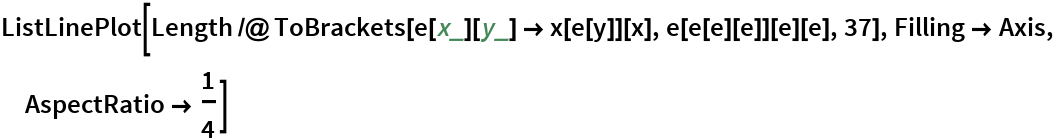Out=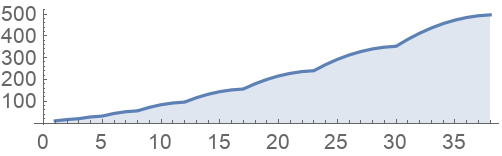Plot the evolution of the symbolic system:

 In:=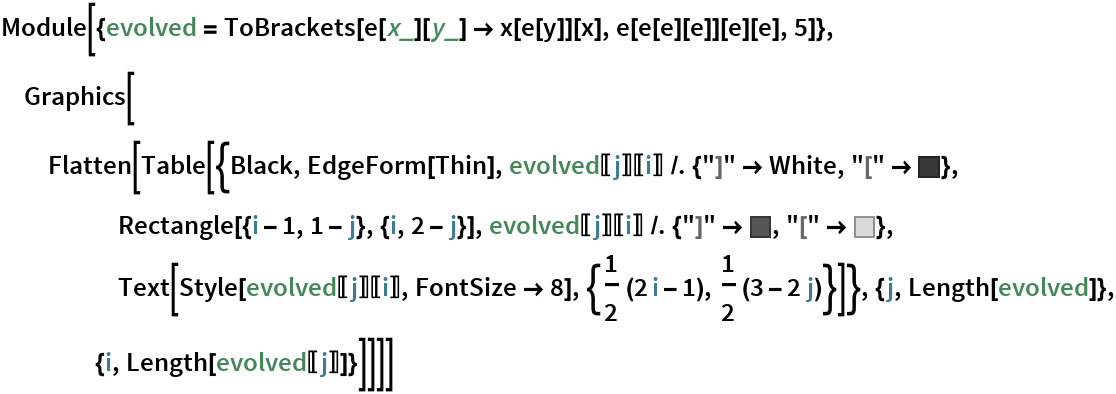Out=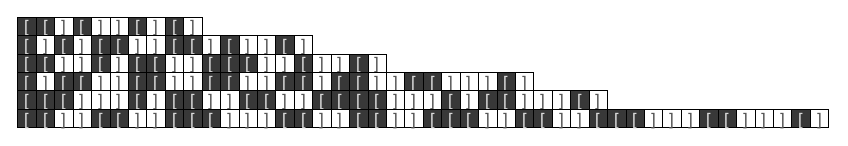In:=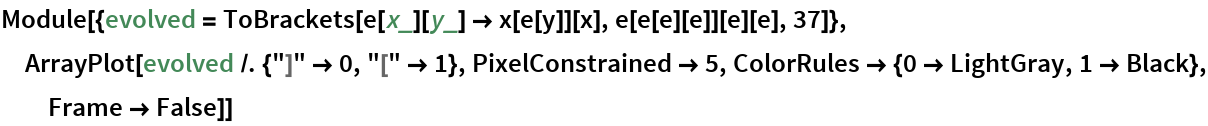Out=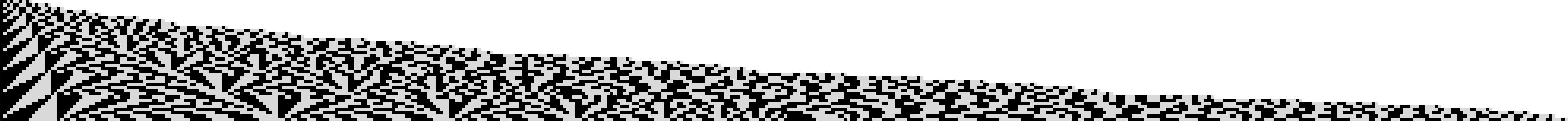## Requirements

Wolfram Language 11.3 (March 2018) or above